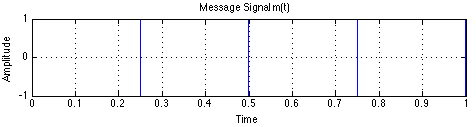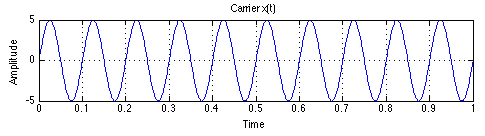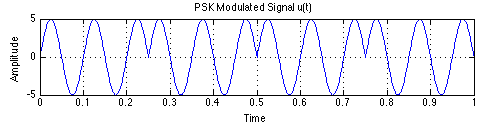# Digital Modulation: using PSK as example on MATLAB

- 2 mins

This semester I had some exposure to digital modulation and learned some techniques applied to the studies of Communications Systems, which we’ll put into practice by using the powerful software MATLAB.

For this tutorial, I’ll pick up the PSK (Phase-Shift Keying). It uses shifts in phase of a signal to represent data. However, if you want and feel comfortable, you can also try it using ASK, FSK or QAM, that along with PSK, form the group of main types of digital modulation widely used in a variety of modern systems, such as cellular networks and so on.

First, let’s suppose we have a message m(t), which is a bit-stream signal, that we want to send through an analog channel. For this example, we consider the frequency of m(t) as 2 Hz. Therefore, we have:

After running this, you should see this image, which represents the message we wanna send:Now we can write the definition for the carrier x(t), a sin() function with frequency of 10 Hz and amplitude A of 5.

Now we can see the carrier x(t) we’re gonna use to modulate our message m(t):Finally, we can plot the modulated (in phase) signal u(t) by just multiplying m(t) by x(t). We’re gonna call u(t) the modulated signal.

Which we can see as:Finally, our message is modulated in PSK, once the phase of the signal does not change while m(t) is equal to 1, being reversed (changing by 180 degrees) when it goes to 0.